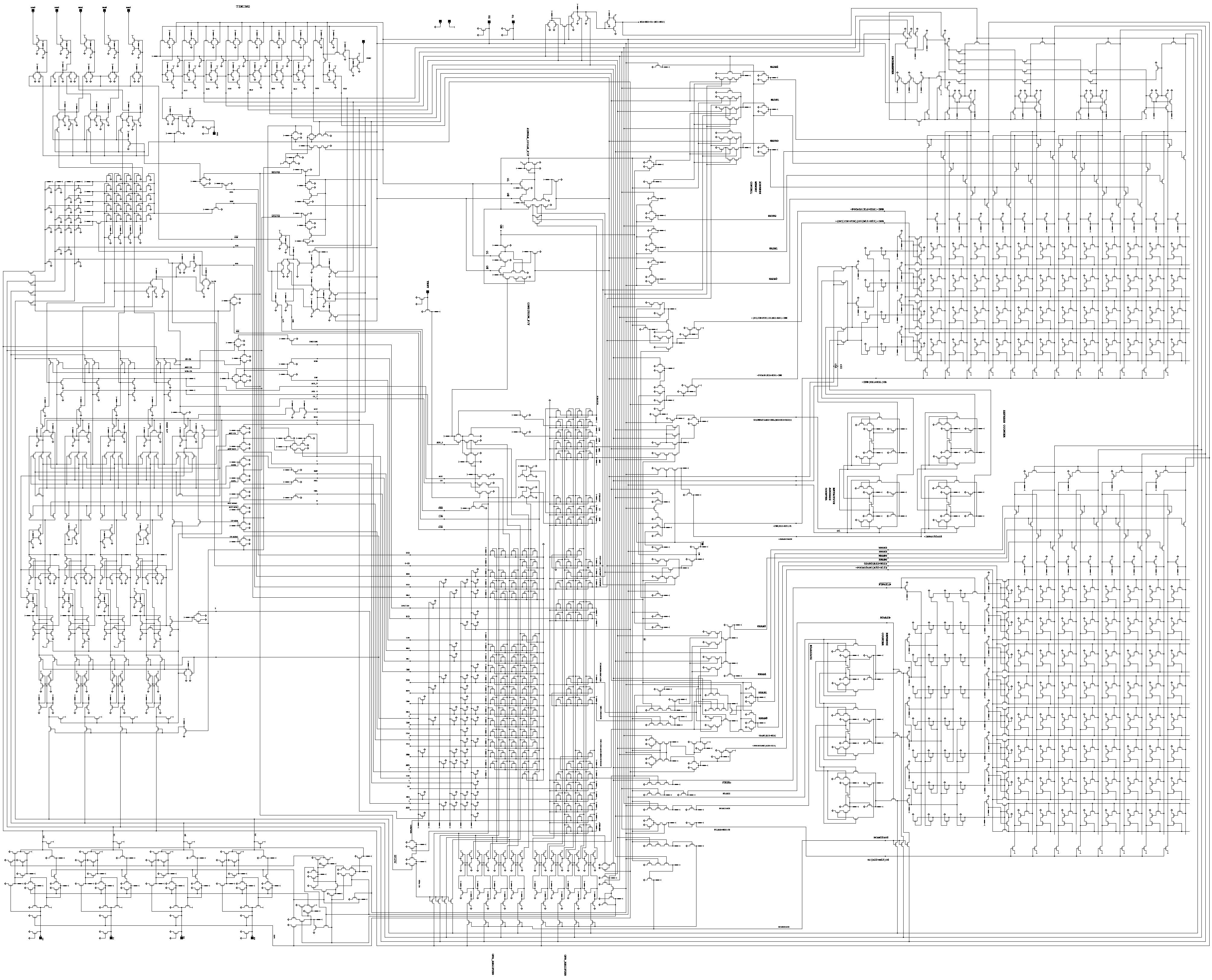# Digital Logic A – Introduction And Logic Gates

Hello readers! In this series, I will be teaching you the basics of Digital Logic. What is Digital Logic? To put it simply, This is the design and creation of components/devices using Logic Gate(s) and Boolean Logic. Every device you use daily, such as your phone, and your computer, use Digital Logic to process data.

This series is made for beginners. However, having a basic understanding of programming or computers would help. At the end of the article, I have linked further reading and watching. With those, you can learn more about the topic

In this part of the series, I will introduce you to the concept of Logic Gates and their usage. I will also give some example gates and their functionality.

## Logic Gates

Logic Gates are at the core of Digital Logic. To put it simply, Logic Gates are electrical devices/circuits that perform boolean logic. Boolean Logic is closely related to Boolean Algebra, the math where operations are done in only two states, True or False.

Since Logic Gates can only have two states, on or off, they can be used to create binary computers. On being 1, Off being 0 (In reality, there is more going on at the electrical level, but that is for another article!). Computer Engineers use Logic Gates to create the components for your devices. A modern CPU may have hundreds of millions of these gates.This is an image of the 4004 processor blueprint, showing every logic gate in the design. It is a simple, 4 bit CPU made in the 1970s. CPU’s are an extremely complex and time-consuming device to create, so do not be worried that it looks confusing!

A logic gate may be represented with a specific symbol. Those symbols will be shown in images for each gate. It is advised to remember them!

## An Example – The AND Gate

The most important Logic Gate (and the simplest) is the AND Gate. Here is a visualized example. This AND Gate has two inputs and one output. Should the two inputs be true, the output is true. However, should both the inputs not be true, the output will remain false or zero. Here is a GIF that demonstrates this behavior.This is a GIF showing how the AND gate functions. In Digital Logic, 1 is considered True or On, and 0 is considered False or Off.

Logic Gates are simply electrical components. It is because of this that they can be combined to create more advanced or abstracted devices. For example, this GIF below explains how using two AND gates, you can create a device that is essentially a three-input AND gate. The same design can be used to make four-input, five-input, and so on.

## Another Example – The NAND Gate

Here is a short example of another gate, the opposite of the AND gate. It is called the NAND Gate. What do you think the gate will do? Accordingly, the opposite of the AND gate! If both inputs are off, or false, the output is on! This GIF will explain the behavior of this gate, with the same format as the AND Gate.As you can see, the output of the gate is ON, or True, if at least one input is off. It is only when both inputs are ON, that the output is false or off.

Using NAND and AND gates, you can create any other Logic Gate or electronic circuit used in today’s devices. However, there are many other Logic Gates that are used commonly, such as OR, NOT, and XOR. We will leave those gates for the next article.

## Conclusion

In this article, we introduced the concept of Digital Logic and Logic Gates, and described two core Gates used in designing digital circuits, the NAND and AND gates. Try them out yourself! There are many online tools, such as LogicLY where you can experiment with the behavior of these gates, and many more, without the hassle of electrics.

In the next article, we will review a couple more Logic Gates, such as OR, NOT, and XOR, as well as introduce Truth Tables, which are a way of keeping track of the behavior of Logic Gates and circuits that use them.

I hope you enjoyed reading this article. If you have any questions or suggestions, please comment below and I will respond! And, like always, make sure to check out other articles on the platform. Thank you for your time.

~Zeek Halkyr

## 3 thoughts on “Digital Logic A – Introduction And Logic Gates”

1.Kvc says:

As always, Your articles and work are truly professional. your ability to quickly grasp any skill at hand will definitely take you ahead in the future.

Thank you for sharing this 🙂

1.ZeekHalkyr says:

I appreciate the feedback!